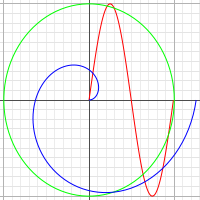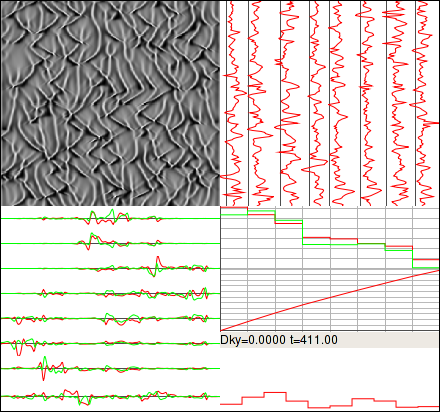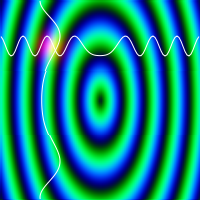# scopemm

scopemm is a simple data plotting package for gtkmm that supports graphs and rasters. Data can be provided using either STL or blitz++. This library was designed to be fast, easy to use, and extensible.

 What you get: Line plots Rasters from a grid of doubles STL interface Optional blitz++ interface Grid and axis displays Manual or auto ranging Mouse events Fast enough to support animated displays What you don't (currently) get: Labels or other annotations Pan or zoom controls 3D plots Points, errorbars, etc.

Another option is plotmm which I somehow was not aware of when I wrote this. I think my interface is easier to use, but plotmm has more types of plots and shows axis labels and tic marks.

To retrieve the source code from GIT:

```git clone https://github.com/dstahlke/scopemm.git
```

## Screenshots## Synopsis

```#include <algorithm>
#include <gtkmm/main.h>
#include <gtkmm/window.h>
#include <math.h>

#include "scopemm.h"

int main(int argc, char *argv[]) {
Gtk::Main kit(argc, argv);

Gtk::Window win;
win.set_title("Test");

scopemm::PlotCanvas plot;

plot.setXAutoRange()
.setYAutoRange()
.setDrawAxes(true)
.setDrawGrids(true);
//plot.setSwapAxes();

scopemm::PlotTrace t1;
scopemm::PlotTrace t2;
scopemm::PlotTrace t3;
t1.setColor(1, 0, 0);
t2.setColor(0, 1, 0);
t3.setColor(0, 0, 1);

const int nsamps = 100;

std::vector<double> ypts;
for(int i=0; i<nsamps; i++) {
double theta = double(i) / (nsamps-1) * 2.0 * M_PI;
ypts.push_back(sin(theta));
}
//t1.setYData(std::make_pair(ypts.begin(), ypts.end()));
t1.setYData(ypts);

std::vector<float> xpts;
ypts.clear();
for(int i=0; i<nsamps; i++) {
double theta = double(i) / (nsamps-1) * 2.0 * M_PI;
// The call to setYData above implicitly used an X range
// of [0, nsamps-1].  We multiply X by nsamps here in order
// to occupy the same range.
xpts.push_back(cos(theta) * nsamps);
ypts.push_back(sin(theta));
}
//t2.setXYData(
//	std::make_pair(xpts.begin(), xpts.end()),
//	std::make_pair(ypts.begin(), ypts.end())
//);
t2.setXYData(xpts, ypts);

std::vector<std::pair<double, double> > xypts;
for(int i=0; i<nsamps; i++) {
double theta = double(i) / (nsamps-1) * 2.0 * M_PI;
// The call to setYData above implicitly used an X range
// of [0, nsamps-1].  We multiply X by nsamps here in order
// to occupy the same range.
double x = 0.2 * theta * cos(theta) * nsamps;
double y = 0.2 * theta * sin(theta);
xypts.push_back(std::make_pair(x, y));
}
//t3.setXYData(std::make_pair(xypts.begin(), xypts.end()));
t3.setXYData(xypts);